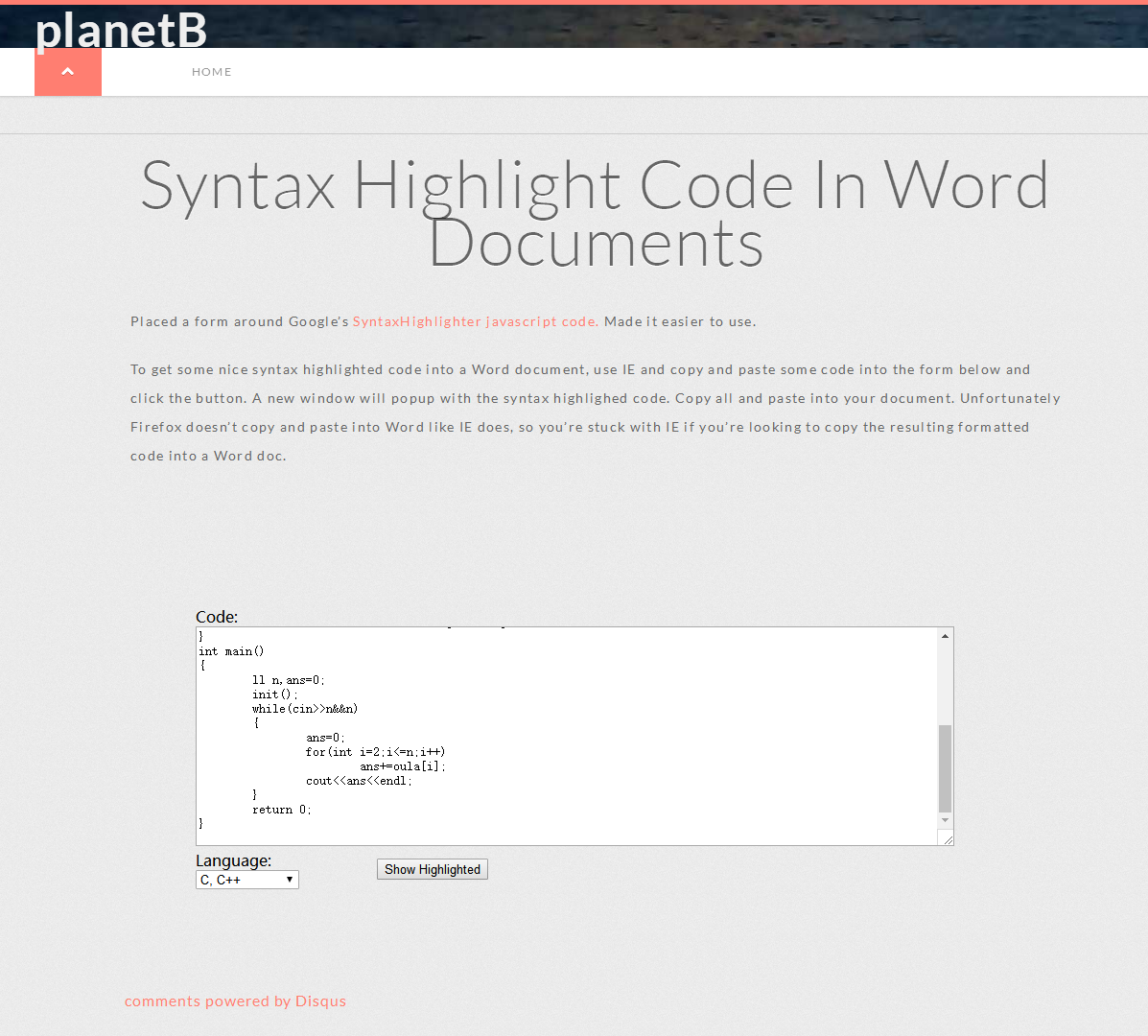# http://www.planetb.ca/syntax-highlight-word生成的代码窗体复制粘贴到word上就好啦。

## 原【欧拉函数模板题】【前缀和】FareySequence

Problem Description

The Farey Sequence Fn for any integer n with n >= 2 is the set of irreducible rational numbers a/b with 0 < a < b <= n and gcd(a,b) = 1 arranged in increasing order. The first few are
F2 = {1/2}
F3 = {1/3, 1/2, 2/3}
F4 = {1/4, 1/3, 1/2, 2/3, 3/4}
F5 = {1/5, 1/4, 1/3, 2/5, 1/2, 3/5, 2/3, 3/4, 4/5}
You task is to calculate the number of terms in the Farey sequence Fn.

Input

There are several test cases. Each test case has only one line, which contains a positive integer n (2 <= n <= 10<sup>6</sup>). There are no blank lines between cases. A line with a single 0 terminates the input.

Output

For each test case, you should output one line, which contains N(n) —- the number of terms in the Farey sequence Fn. <b

Sample Input

2

3

4

5

0

Sample Output

1

3

5

9

## 思路：由a / b,gcd(a,b)=1知，当前f(n)是在f（n – 1）的基础上加上以n为分母，与n互质的数为分子的分数，所以f（n）比f（n – 1）增加了1~n内与n互质的数的个数，现在题意就很明显了，就是要求1-N的欧拉函数之和。

#include <iostream>
using namespace std;
typedef long long ll;
const int MAX=1000005;
int oula[MAX];
void init()
{
oula=1;
for(int i=2;i<MAX;i++)
oula[i]=i;
for(int i=2;i<MAX;i++)
if(oula[i]==i)
for(int j=i;j<MAX;j+=i)
oula[j]=oula[j]/i*(i-1);
}
int main()
{
ll n,ans=0;
init();
while(cin>>n&&n)
{
ans=0;
for(int i=2;i<=n;i++)
ans+=oula[i];
cout<<ans<<endl;
}
return 0;
}


## 原SDAU暑假训练第12天做题（2018810）

PS:做题速度大佬们一个比一个快，，菜鸡感到压力很大

## 【leetcode458】【规律题】【突破性思维】可怜的小猪

【leetcode458】【规律题】【突破性思维】可怜的小猪

# 分析

00     01     02     03      04

10     11     12     13      14

20     21     22     23      24

30     31     32     33      34

40     41     42     43      44

java代码：

class Solution {
public int poorPigs(int buckets, int minutesToDie, int minutesToTest) {

if(buckets == 1) return 0;

int w = minutesToTest / minutesToDie + 1;
int re = 1;
while(Math.pow(w,re) < buckets)
re ++;

return re;
}
}

class Solution {
public:
int poorPigs(int buckets, int minutesToDie, int minutesToTest) {
if(minutesToTest < minutesToDie)
return 0;
return ceil(log(buckets) / log(floor(minutesToTest/minutesToDie) + 1));
}

};

## 题目不严谨，有可能只需一只猪就可以判断1000个桶